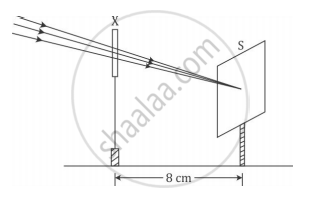Share

# A student used a device (X) to obtain/focus the image of a well illuminated distant building on a screen (S) as shown below in the diagram. Select the correct statement about the device (X). - CBSE Class 10 - Science

ConceptReflection of Light Spherical Mirrors

#### Question

A student used a device (X) to obtain/focus the image of a well illuminated distant building on a screen (S) as shown below in the diagram. Select the correct statement about the device (X).(A) This device is a concave lens of focal length 8 cm.

(B) This device is a convex mirror of focal length 8 cm.

(C) This device is a convex lens of focal length 4 cm.

(D) This device is a convex lens of focal length 8 cm.

#### Solution

(D) This device is a convex lens of focal length 8 cm.

The incident rays after passing through the lens converge at the focus. So, the device 'X' is a converging or a convex lens. The distance between the lens and the screen gives the focal length of the lens.

Is there an error in this question or solution?

#### Video TutorialsVIEW ALL 

Solution A student used a device (X) to obtain/focus the image of a well illuminated distant building on a screen (S) as shown below in the diagram. Select the correct statement about the device (X). Concept: Reflection of Light - Spherical Mirrors.
S